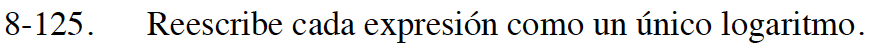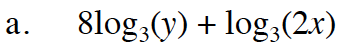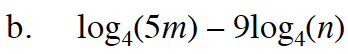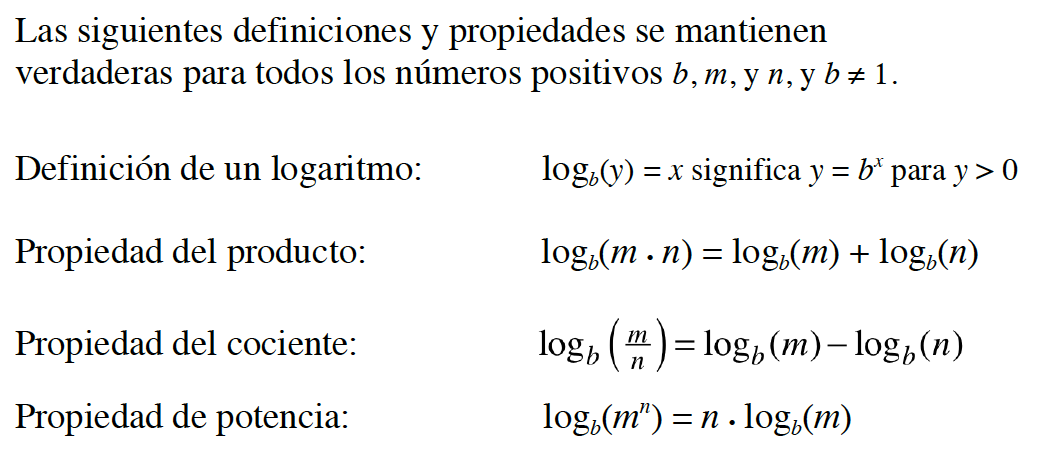8-125.

### Missing problem for Book int3s => Chapter Ch8 => Lesson 8.3.2 => Problem 8-125

Created from orphaned homework help problem$\text{log}_4\left(\frac{5m}{n^9}\right)$